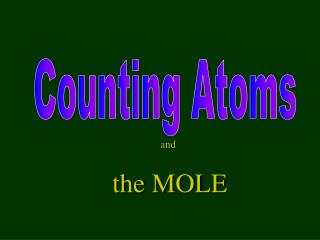DownloadDownload Presentationand the MOLE

# and the MOLE

Download Presentation## and the MOLE

- - - - - - - - - - - - - - - - - - - - - - - - - - - E N D - - - - - - - - - - - - - - - - - - - - - - - - - - -
##### Presentation Transcript

1. and the MOLE

2. Using Mass to Count Things • Atoms are so tiny it is impossible to count # atoms in only 1 gram of matter use mass to “count” atoms

3. Counting by mass • You have a pile of nails that weighs 1227 g • One nail has a mass of 0.450 grams • How many nails are in the pile? 1227g = 2726.6 nails 0.450 g per nail [Let’s call it 2730 nails]

4. What is a mole? Not this kind of mole!

5. The MOLE • Unit used to “count” # atoms • Count-by-mass technique • Defined: # atoms in 12.0 grams of C-12 • This is the STANDARD! • 12.0 g of C-12 has 6.0221415 X 1023 atoms

6. The MOLE • 1 mole of any element has 6.02 X 1023 particles • This is a really big number – It’s so big because atoms are really small!

7. 6.02 X 1023 = Avogadro’s Number • One mole of water = 6.02 X 1023 water molecules • One mole of gorillas is 6.02 X 1023 gorillas • One mole of He atoms is 6.02 X 1023 He atoms • One mole of anything is 6.02 X 1023 of that thing

8. Similar Words • Pair: 1 pair of shoelaces = 2 shoelaces • Dozen: 1 dozen oranges = 12 oranges • Gross: 1 gross of spider rings = 144 rings • Ream: 1 ream of paper = 500 sheets paper • Mole: 1 mole of Na atoms = 6.02 X 1023 Na atoms

9. Equalities 1 mole of C-12 = 12.0 grams of C-12 = 6.02 X 1023 atoms of C-12

10. 0.5 mole of C-12 = 6.0 grams of C-12 = 3.01 X 1023 atoms of C-12

11. 0.25 mole of C-12 = 3.0 grams of C-12 = 1.50 X 1023 atoms of C-12 Equalities 1 mole C-12 = 12.0 g C-12 = 6.02 x 1023 atoms C-12

12. 2 moles of C-12 = 24.0 grams of C-12 = 12.04 X 1023 atoms C-12 [= 1.204 X 1024 atoms C-12] Can this help with other elements? Equalities 1 mole C-12 = 12.0 g C-12 = 6.02 X 1023 atoms C-12

13. What is the mass of 1 mole of Mg (magnesium)? 1 mole of Mg = 24 grams

14. MOLAR mass for any element: molar mass of any element = atomic mass in g/mole 1 mole Ne = 20 g/mol [= 6.02 X 1023 atoms] 1 mole Ar = 40 g/mol [= 6.02 X 1023 atoms] 1 mole Kr = 84 g/mol [= 6.02 X 1023 atoms]

15. Simple Mole Map

16. No. of Grams by No. of Moles  by by No. of Liters No. of Particles formula mass x by x by 6.02 x 1023 particles/mol x by 22.4 L/mol